# Angular boundary value

(diff) ← Older revision | Latest revision (diff) | Newer revision → (diff)

boundary value along a non-tangential path

The value associated to a complex function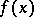defined in the unit disc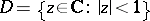at a boundary point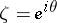, equal to the limitof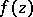on the set of points of the angular domain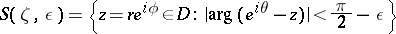under the condition that this limit exists for all,, and hence does not depend on. The term is sometimes applied in a more general sense to functionsgiven in an arbitrary (including a higher-dimensional) domain; forone takes the intersection withof an angular (or conical) domain with vertex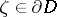, with axis normal to the boundary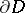atand with angle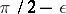,.

How to Cite This Entry:
Angular boundary value. Encyclopedia of Mathematics. URL: http://encyclopediaofmath.org/index.php?title=Angular_boundary_value&oldid=12519
This article was adapted from an original article by E.D. Solomentsev (originator), which appeared in Encyclopedia of Mathematics - ISBN 1402006098. See original article